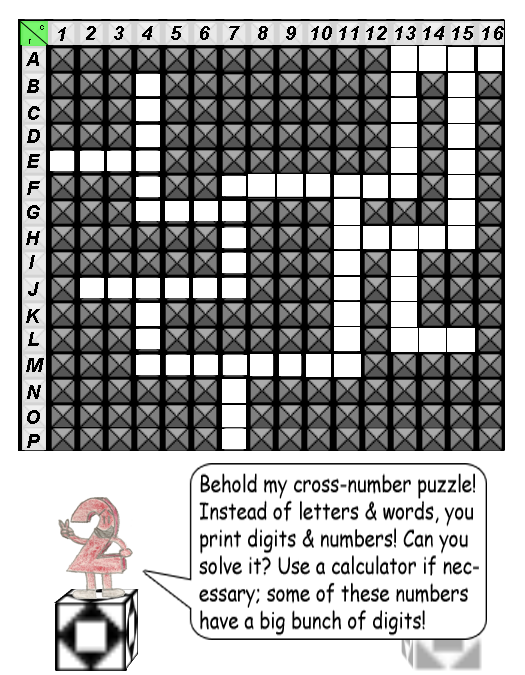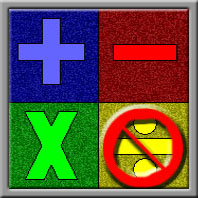# Mr. Two's Cross-Number Puzzle

#### Difficulty: MediumThe answers to the math problems below are what you have to print in the blank squares. Each blank square represents a single digit in the number of each correct answer to a math problem. Count the number of squares(up-to-down or left-to-right) & you will know how many digits the correct number should have.

As you can see, there are 16 rows & 16 columns; the letters(A-P) stand for the rows, & the numbers(1-16) stand for the columns. The math problems are marked by row letter first, and then column number; for example, a problem marked "A1" means you must print the digit in the number's largest column first in that blank square.

The up-to-down math problems are in the left column, & the left-to-right math problems are in the right column of the table below. You will only get math problems in arithmetic & pre-algebra; division is the only arithmetic operation that has been excluded from this puzzle.P.S.: Remember that pre-algebra rule: "Please Excuse My Dear Aunt Sally!"

### Math Problems:

 Up-to-Down Left-to-Right B4: 10^6 - 1 = ? E1: 413 × 3 = ? F7: 144 × 100 + 14 × 2 = ? G4: 9,000 + 200 + 74 = ? J4: 25 × 101 = ? F7: 800 + 175 × 10^4 = ? M7: 1571 × 4 = ? H11: 262 × 125 = ? F11: 8 × 10^7 + 33 × 10^5 + 55 = ? J2: 1,572,222 - 999,754 = ? A15: 6,990 + 6,002 × 10^4 = ? A13: 1,234 × 2 = ? H13: 3^2 × 8,441 = ? L13: 103 × 9 = ? A13: 250 × 10^3= ? M4: 25(558,422 + 1,480,085) = ?